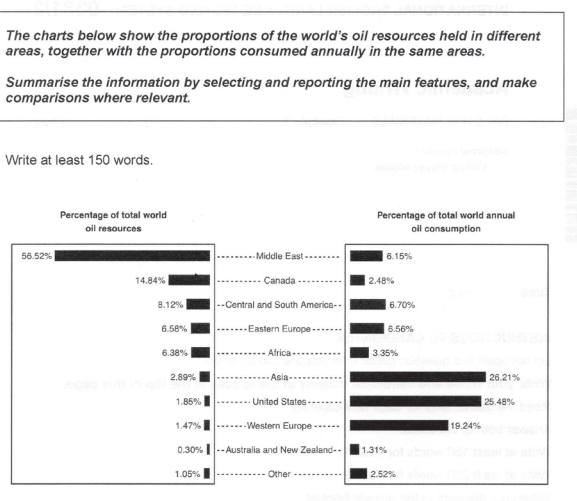Mathematical Calculations for Task 1?

edited April 2
Consider the following IELTS Task 1 question:Now, read part of a student's response:

On the other hand, Asia which consumes 26.21% of oil resources contributes only about one ninth of this total.

What's wrong here?

The problem is the mathematical calculation used.  If you make complicated mathematical calculations in your IELTS Task 1 answer, you'll scare the examiner away.

Avoid (complicated) mathematical calculations.  In general, the following words are suitable for making comparisons:

• double
• twofold
• twice
• three/four/five times
• half
• much more
• dramatically more/less
Write some sentences using the comparison language above about the Task 1 horizontal bar chart in the box below

Tagged: